How to Implement the Omron Host Link Protocol Part 2 – VBA

In Part 1, we used VB6 to communicate from the computer to the PLC. We will now use Visual Basic for Applications (VBA) to accomplish the same task. NETComm will be the serial driver and Excel will be the program that we will use. Using Excel we will have access to the Excel Object Model so we can utilize worksheets, ranges, etc.

Please refer to Part 1 (How to Implement the Omron PLC Host Link Protocol) for the details of the wiring of the serial port and protocol sequence.

The first step in using VBA is to download and register NETComm.ocx. To use serial communications with VBA you must register the NETComm.ocx driver.

Call up a dos prompt with administrator authority. (Right-click on the cmd.exe program and select run as administrator.)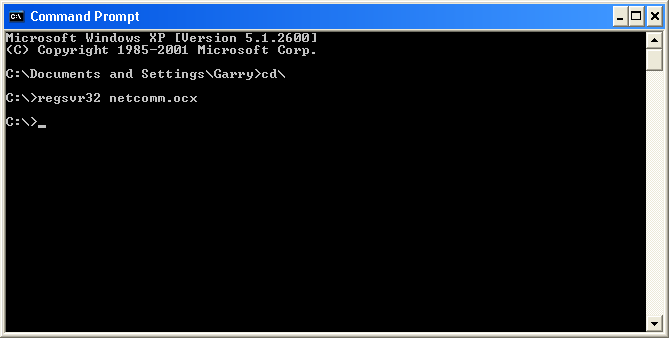Type ‘regsvr32 netcomm.ocx’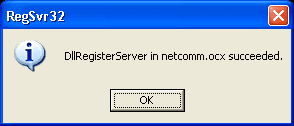An information box will be shown indicating that the NETComm.ocx was registered successfully.

Now open Excel and make the following on Sheet 1.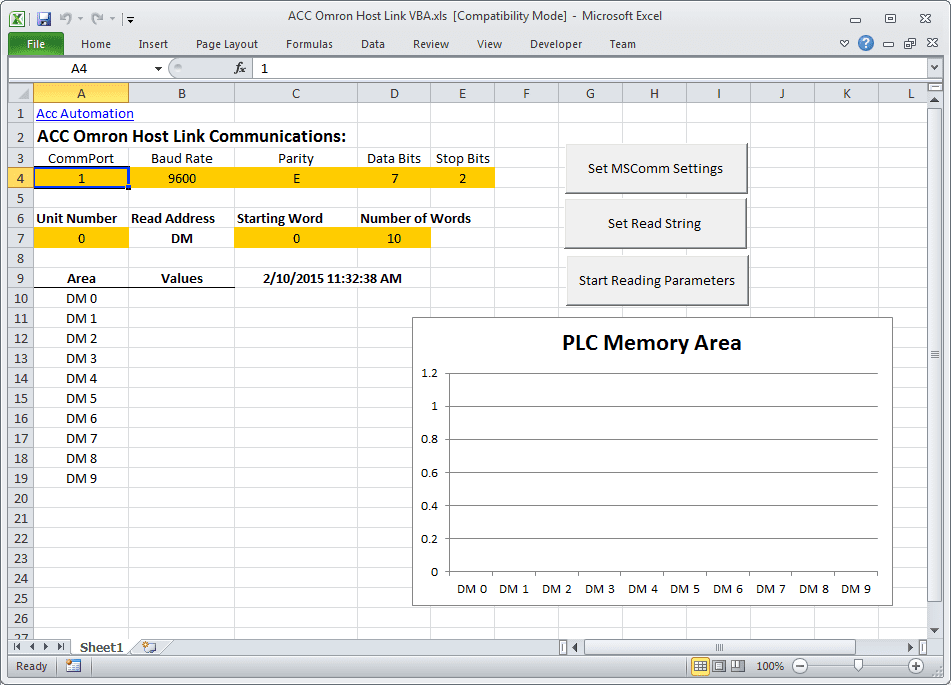Select Developer and then Visual Basic.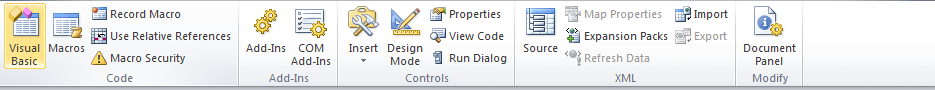If you do not have the Developer option to select, then do the following:
Select File : Options: Customized Ribbon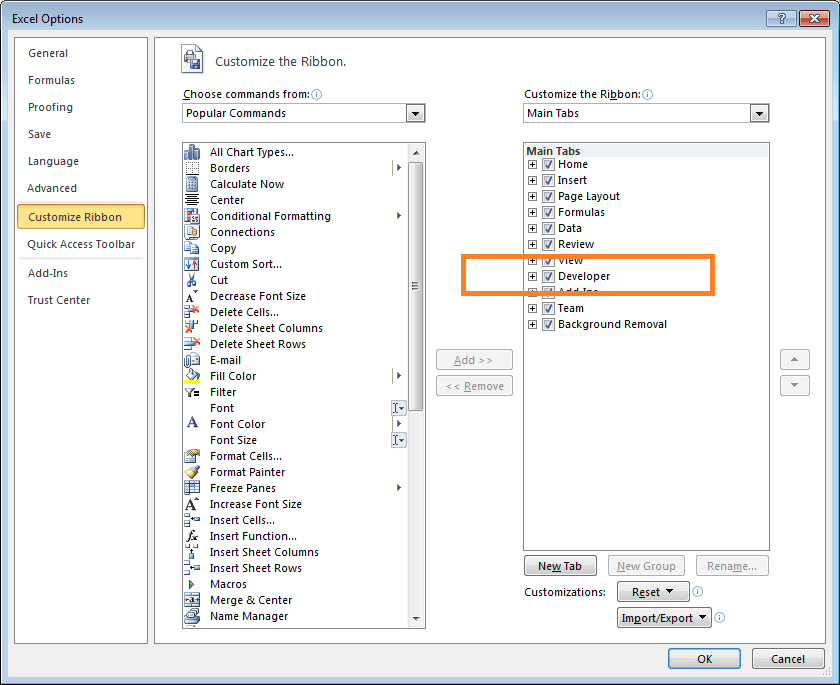Select Developer and hit OKVBA – Visual Basic for Applications will open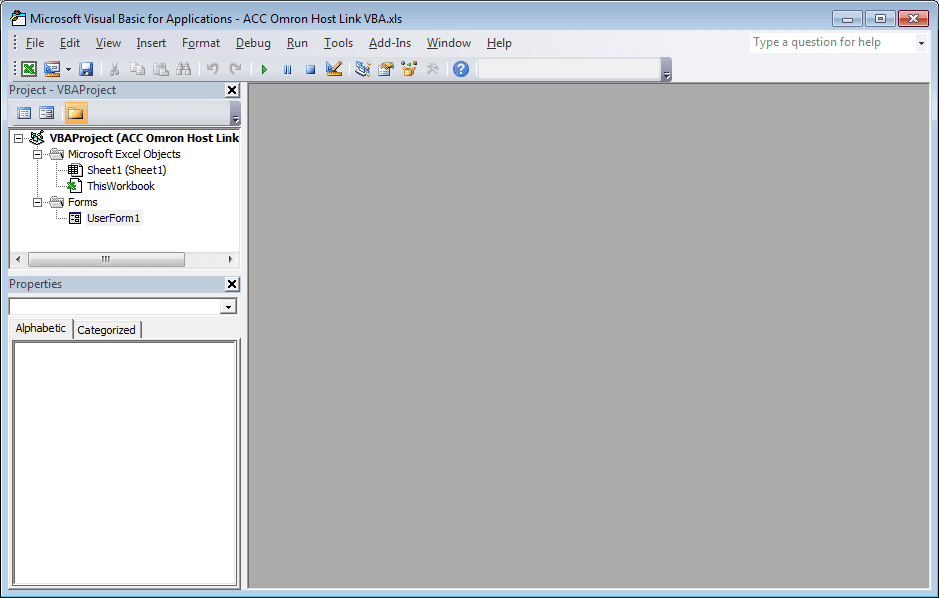Add NETComm1 to UserForm1. If it is not on your Toolbar, then select additional controls and select NETCommOCX.NETComm. Press the OK. Then drag this control onto your form.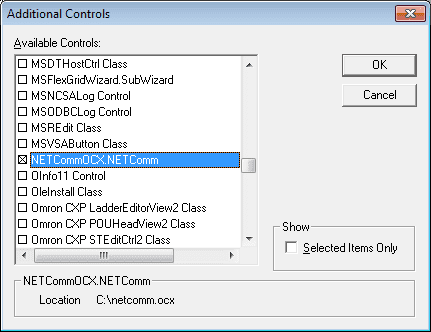Create three command buttons. This can be done by going to Design Mode,  and under the Insert menu you can select Command Buttons.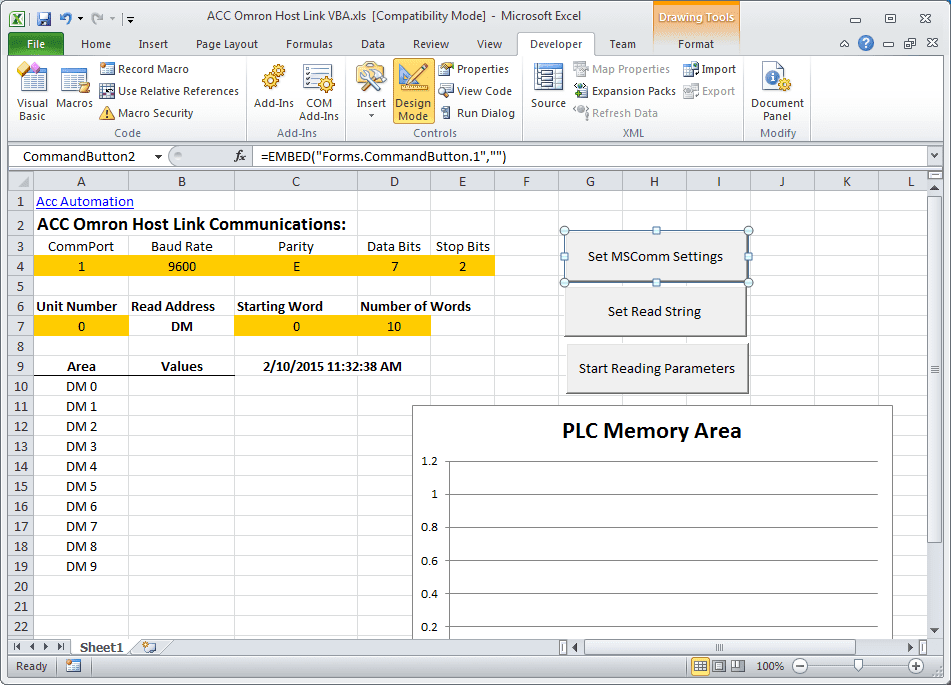Here is the entire code for the application:

```Private T\$ ' Modual Scope for variable
Private NumWords
Private startcycle```
```Private Sub CommandButton1_Click()
If NumWords = "" Then
Exit Sub
End If
If startcycle = 0 Then
startcycle = 1
CommandButton1.BackColor = "&H0000FF00"
Else
startcycle = 0
CommandButton1.BackColor = "&H8000000F"
UserForm1.NETComm1.PortOpen = False ' Close Communication Port
Exit Sub
End If
UserForm1.NETComm1.PortOpen = True ' Open Communication Port
Application.EnableEvents = True
Do
T\$ = TRead\$ ' Reset the Transmitted Information
charreturn = 11 + (NumWords * 4) ' Determine the return characters
GoSub FCS ' Checksum
GoSub communicate ' Get informaiton

'Check returned information and Display
If Mid(rxd\$, 6, 2) = "00" And (Len(rxd\$)) >= charreturn Then
For x = 1 To Sheets("Sheet1").Range("D7")
Sheets("Sheet1").Range("B" & x + 10 - 1) = Mid(rxd\$, x * 4 + 4, 4)
Next 'x
End If```
```    DoEvents ' Do other tasks
'Update the date and time
Sheets("Sheet1").Range("C09") = Format(Date, "YYYY/MM/DD") + "    " + Format(Time, "HH:MM:SS")
Loop While startcycle = 1```
`If startcycle = 0 Then Exit Sub`
```communicate:
rxd\$ = ""
Buffer = T\$ + FCS\$ + "*" + Chr\$(13)
UserForm1.NETComm1.Output = Buffer
Time1 = Now
Timeout = Now + TimeValue("0:00:02")
Do
If Timeout <= Time1 Then GoTo timeoutcom
DoEvents
Time1 = Now()
Loop Until UserForm1.NETComm1.InBufferCount >= charreturn
rxd\$ = UserForm1.NETComm1.InputData
fcs_rxd\$ = Left((Right(rxd\$, 4)), 2)
If Left(rxd\$, 1) = "@" Then
T\$ = Mid(rxd\$, 1, (Len(rxd\$) - 4))
ElseIf Mid(rxd\$, 2, 1) = "@" Then
T\$ = Mid(rxd\$, 2, (Len(rxd\$) - 5))
rxd\$ = Mid(rxd\$, 2, (Len(rxd\$) - 1))
End If
GoSub FCS
If FCS <> fcs_rxd\$ Then
rxd\$ = ""
End If
clearbuffer\$ = UserForm1.NETComm1.InputData
Return```
```FCS:
L = Len(T\$)
A = 0
For J = 1 To L
TJ\$ = Mid\$(T\$, J, 1)
A = Asc(TJ\$) Xor A
Next J
FCS\$ = Hex\$(A)
If Len(FCS\$) = 1 Then FCS\$ = "0" + FCS\$
Return```
```timeoutcom:
If startcycle = 0 Then Exit Sub
clearbuffer\$ = UserForm1.NETComm1.InputData
rxd\$ = ""
Return```
`End Sub`
```Private Sub CommandButton2_Click()
If startcycle = 0 Then
UserForm1.NETComm1.CommPort = Sheets("Sheet1").Range("A4")
UserForm1.NETComm1.Settings = Sheets("Sheet1").Range("B4") & "," & Sheets("Sheet1").Range("C4") & "," & Sheets("Sheet1").Range("D4") & "," & Sheets("Sheet1").Range("E4")
MsgBox UserForm1.NETComm1.Settings
Else
MsgBox "Stop Reading Parameters to Set MSComm"
End If
End Sub```
```Private Sub CommandButton3_Click()
If startcycle = 0 Then
Unit = Sheets("Sheet1").Range("A7")
If Len(Unit) < 2 Then Unit = "0" & Unit
Do
End If
NumWords = Sheets("Sheet1").Range("D7")
Do
If Len(NumWords) < 4 Then
NumWords = "0" & NumWords
End If
Loop Until Len(NumWords) = 4
T\$ = "@" & Unit & "RD" & StartADD & NumWords
MsgBox T\$
For x = 1 To Sheets("Sheet1").Range("D7")
Sheets("Sheet1").Range("A" & x + 10 - 1) = "DM " & Sheets("Sheet1").Range("C7") + x - 1
Sheets("Sheet1").Range("B" & x + 10 - 1) = ""
Next 'x
For x = Sheets("Sheet1").Range("D7") To 200
Sheets("Sheet1").Range("A" & x + 10) = ""
Sheets("Sheet1").Range("B" & x + 10) = ""
Next 'x
Else
End If
End Sub```
`Private Sub Worksheet_SelectionChange(ByVal Target As Range)`
`End Sub`

Note: The Chart on Sheet1 is just a selection of the first ten DM areas and insert a bar graph.

Running the program produces the following information: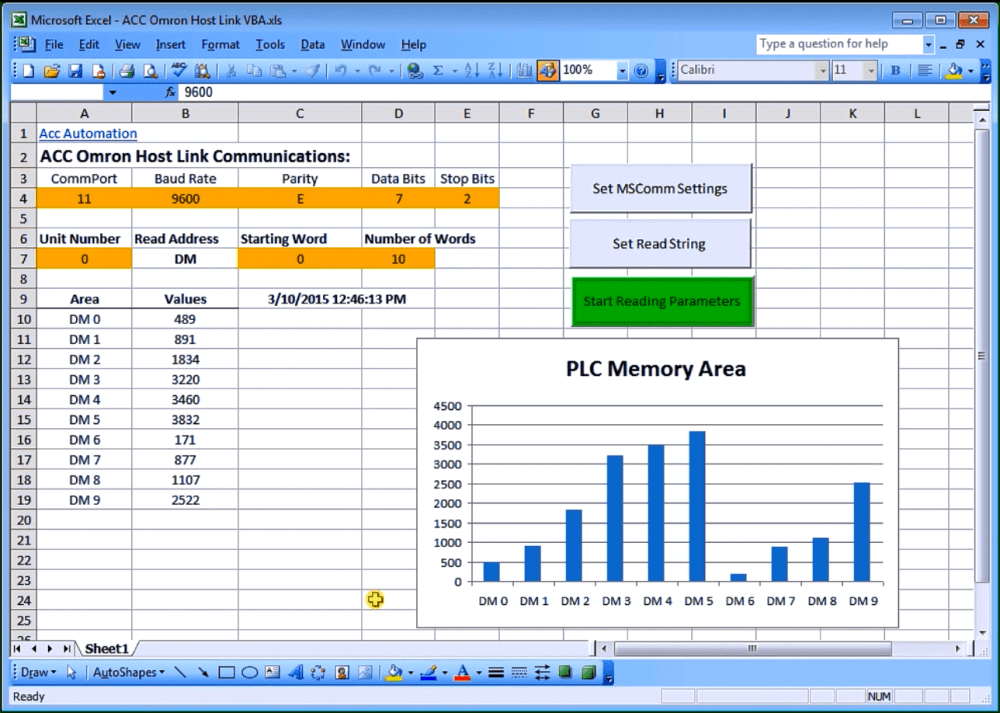When you open the file it will warn you about macros. This is the VBA application. Press ‘Enable Macros’.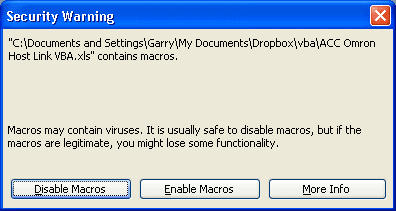When changing the parameters on the screen, you will also get a warning about Active X. This is the NETComm.ocx which was registered above. Press ‘OK’ to run the application.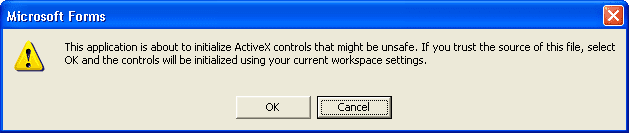Excel – Conditional Movement of Data

Watch on YouTube: How to Implement the Omron Host Link Protocol Part 2 – VBA
Thank you,
Garry

If you’re like most of my readers, you’re committed to learning about technology. Numbering systems used in PLC’s are not difficult to learn and understand. We will walk through the numbering systems used in PLCs. This includes Bits, Decimal, Hexadecimal, ASCII and Floating Point.

To get this free article, subscribe to my free email newsletter.

Use the information to inform other people how numbering systems work. Sign up now.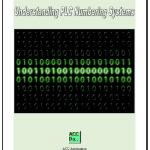2 thoughts on “How to Implement the Omron Host Link Protocol Part 2 – VBA”

1.Satvir Singh says:

HI,

I was looking around the website and came across this article “How to Implement the Omron PLC Host Link Protocol” and Garry you mentioned that you are able to give a copy of the program. Could I possible obtain this copy, as currently I’m working on a project and I having difficulties in getting a suitable VB example that would let send commands to Cable LC-RS9 RS232 9 pin for IBM PCXT for a weighting scale (SAG105 SAG105 mettler toledo ). Maybe this software is able to accomplish this. Unless you provide something that might be more related.

Many thanks
Satvir Singh

1.garrys says:

Hi Satvir,

I have sent you a separate email with a program link to download. The following is the manual location for the Mettler Toledo scale communications.
Testing the cable sounds like the biggest challenge. You could use Hype Terminal on the PC which will read the serial information coming in from the port.
Hope this helps you out Satvir.
Garry